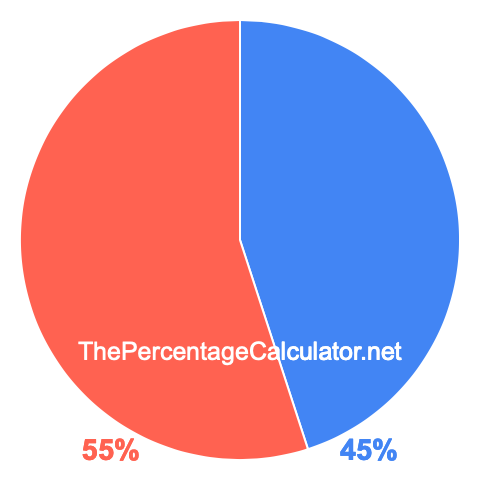Pie Chart showing 45 percent

Here we will give you step-by-step instructions on how to create a pie chart showing 45 percent. (This is also sometimes referred to as a pie chart showing 45 percentage.)

Step 1) Calculate the degrees
To create a pie chart showing 45 percent, you first need to calculate 45% of 360, because there are 360 degrees in a circle (aka pie). 45 percent of 360 is 162 degrees.

Step 2) Outline 45 percent of the circle
For this part, you need a protractor, which is a tool to measure angles. Use your protractor to outline 0 degrees to 162 degrees on a circle.

Step 3) Color the area
This is the final step. Color the area you outlined in Step 2 so you can clearly see how much 45 percent is of the pie. We colored our outlined area in blue. Here is our result so you can compare it to yours:The area shaded in red on our pie chart above is the remaining part of the whole pie and is calculated by subtracting 45% from 100%.

Note that when you follow the steps above, it does not matter how small or large you make the circle. The protractor will make sure that the answer will be correct regardless of the circle size as long as you calculated the degrees correctly.

Pie Chart Percent Generator
Here you can generate a pie chart for another percentage.

Pie Chart showing 45.01 percent
Go here for the next pie chart we created for you.